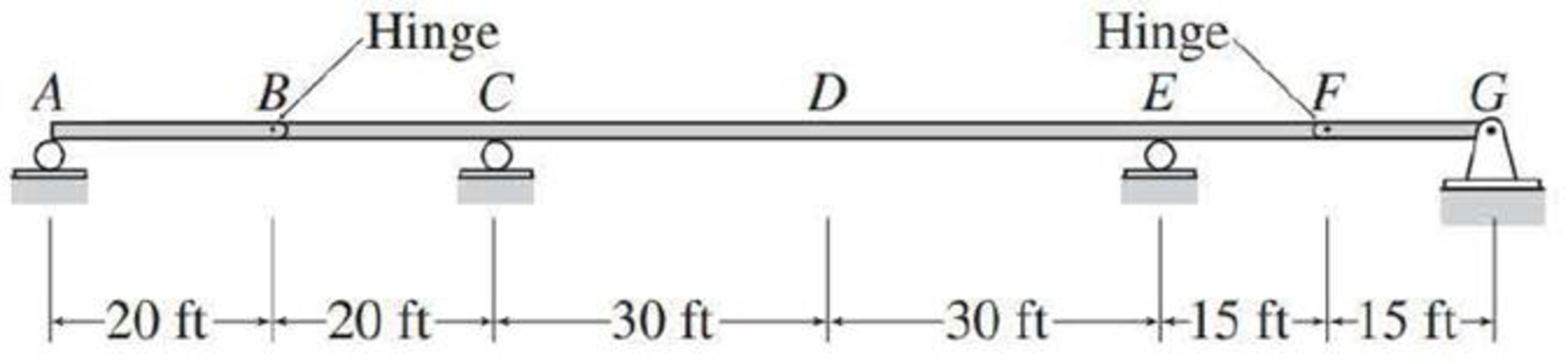# Draw the influence lines for the vertical reactions at supports A , C , E , and G of the beams shown in Figs. P8.23 and P8.24. FIG. P8.23, P8.25

#### Solutions

Chapter
Section
Chapter 8, Problem 23P
Textbook Problem
81 views

## Draw the influence lines for the vertical reactions at supports A, C, E, and G of the beams shown in Figs. P8.23 and P8.24.FIG. P8.23, P8.25

To determine

Draw the influence lines for the vertical reactions at supports A, C, E and G.

### Explanation of Solution

Calculation:

Use Muller-Breslau's principle.

Remove the roller support at A from the given beam and apply a small displacement Δ. Due to the displacement, the structure released in which the deflected shape of the released structure of the beam is drawn.

Sketch the deflected shape of the released beam when roller support A is removed as shown in Figure 1.

The general shape of the influence line for vertical reaction (Ay) at supports A is the similar of deflected shape.

Sketch the general shape of the influence line for vertical reaction (Ay) at supports A as shown in Figure 2.

Apply 1 k unit load at point A.

Find the numerical value of the influence-line ordinate at A.

Generally the influence line ordinate (AA’) at A is 1 k/k when 1 k load is placed at A.

Therefore, the influence line ordinate is 1 k/k at A and 0 at all other points.

Sketch the influence line diagram for the vertical reactions at support A as shown in Figure 3.

Remove the roller support at C from the given beam and apply a small displacement Δ. Due to the displacement, the structure released in which the deflected shape of the released structure of the beam is drawn.

Sketch the deflected shape of the released beam when roller support C is removed as shown in Figure 4.

The general shape of the influence line for vertical reaction (Cy) at supports C is the similar of deflected shape.

Sketch the general shape of the influence line for vertical reaction (Cy) at supports C as shown in Figure 5.

Refer Figure 5.

The influence line ordinate at A, E, and G is 0.

Apply 1 k unit load at point C.

Find the numerical value of the influence-line ordinate at C.

Generally the influence line ordinate (CC’) at C is 1 k/k when 1 k load is placed at C. The value of influence line ordinate in the different points on the beam determined by geometry (similar triangle) of the influence line.

Find the numerical value of influence line ordinate (BB’) at point B using similar triangle method.

BBBE=CCCEBB80=160BB=43

Similarly calculate the influence line ordinate for Cy in different points on beam and summarize the values as in Table 1

 x (ft) Points Influence line ordinate of Cy (k/k) 0 A 0 20 B 43 40 C 1 70 D 12 100 E 0 115 F −14 130 G 0

Sketch the influence line diagram for the vertical reactions at support C as shown in Figure 6.

### Still sussing out bartleby?

Check out a sample textbook solution.

See a sample solution

#### The Solution to Your Study Problems

Bartleby provides explanations to thousands of textbook problems written by our experts, many with advanced degrees!

Get Started

Find more solutions based on key concepts
What do we mean by dimensional homogeneity? Give an example.

Engineering Fundamentals: An Introduction to Engineering (MindTap Course List)

What are the two basic styles of data replication?

Database Systems: Design, Implementation, & Management

What are the three basic operations in cryptography?

Principles of Information Security (MindTap Course List)

Write a query to count the number of customers with a balance of more than 500.

Database Systems: Design, Implementation, & Management

Define an object, and provide three examples.

Systems Analysis and Design (Shelly Cashman Series) (MindTap Course List)

No Matching Port Your uncle has given you a new monitor for your computer. When you attempt to connect it, you ...

Enhanced Discovering Computers 2017 (Shelly Cashman Series) (MindTap Course List)

If your motherboard supports ECC DDR3 memory, can you substitute non-ECC DDR3 memory?

A+ Guide to Hardware (Standalone Book) (MindTap Course List)